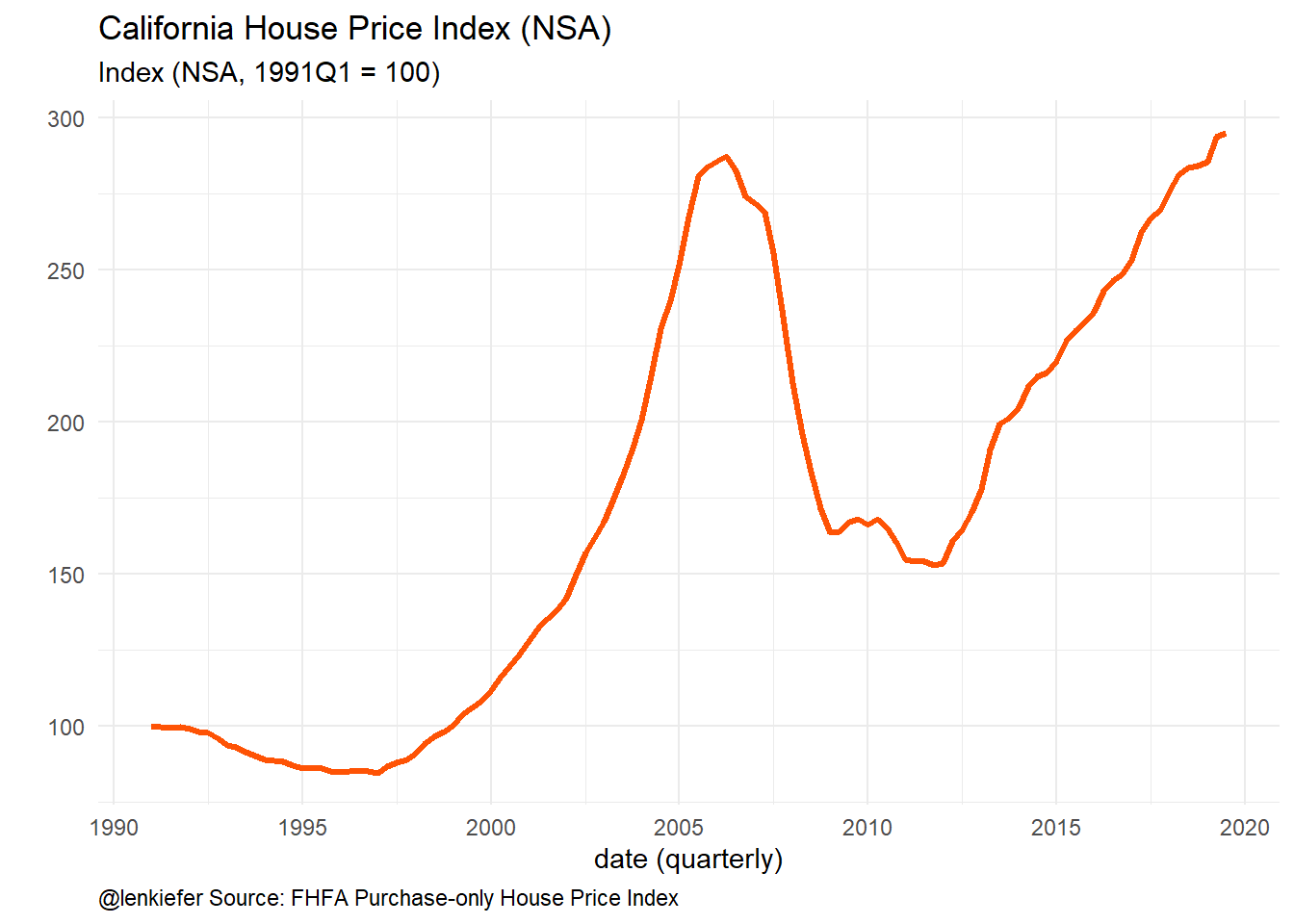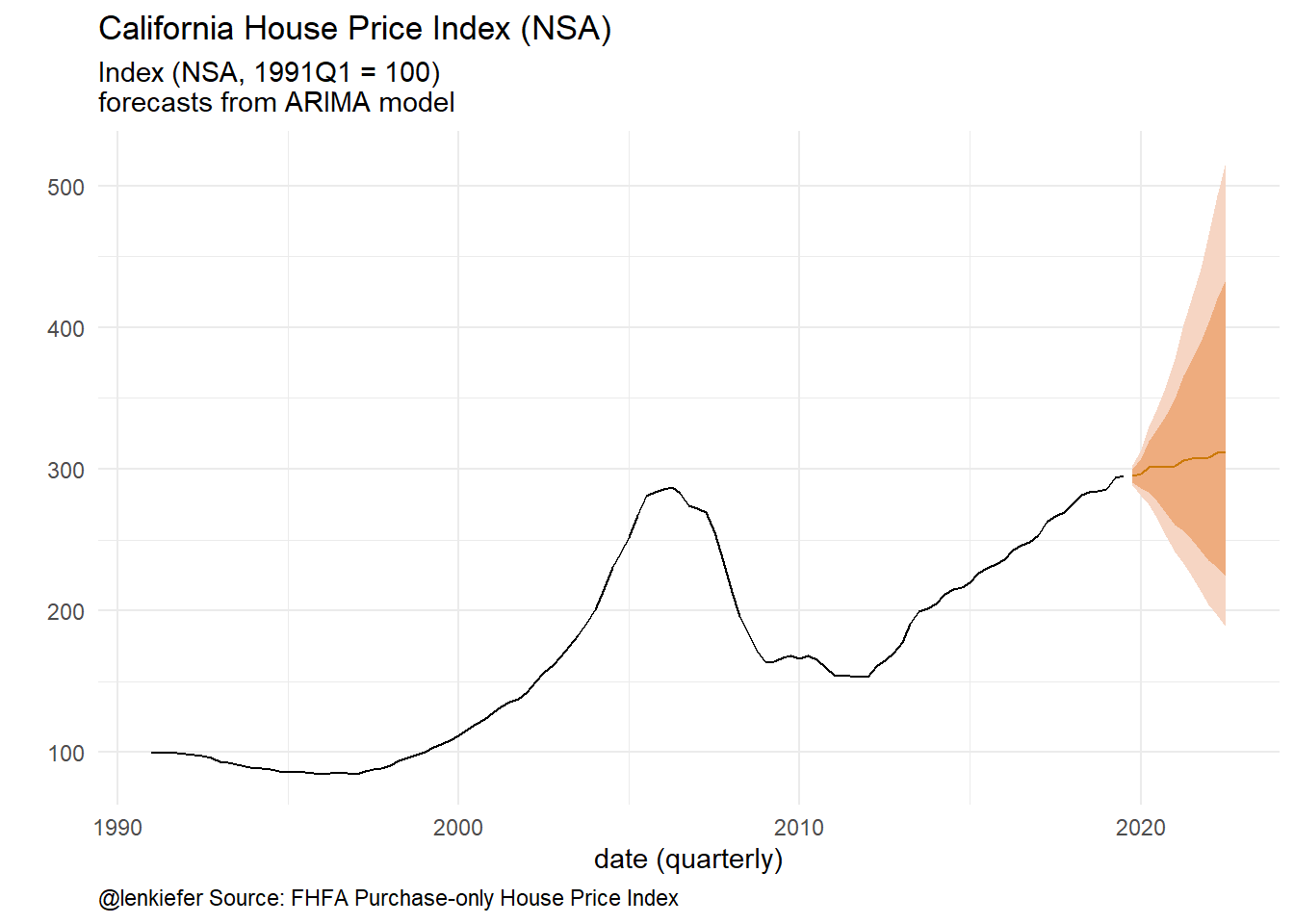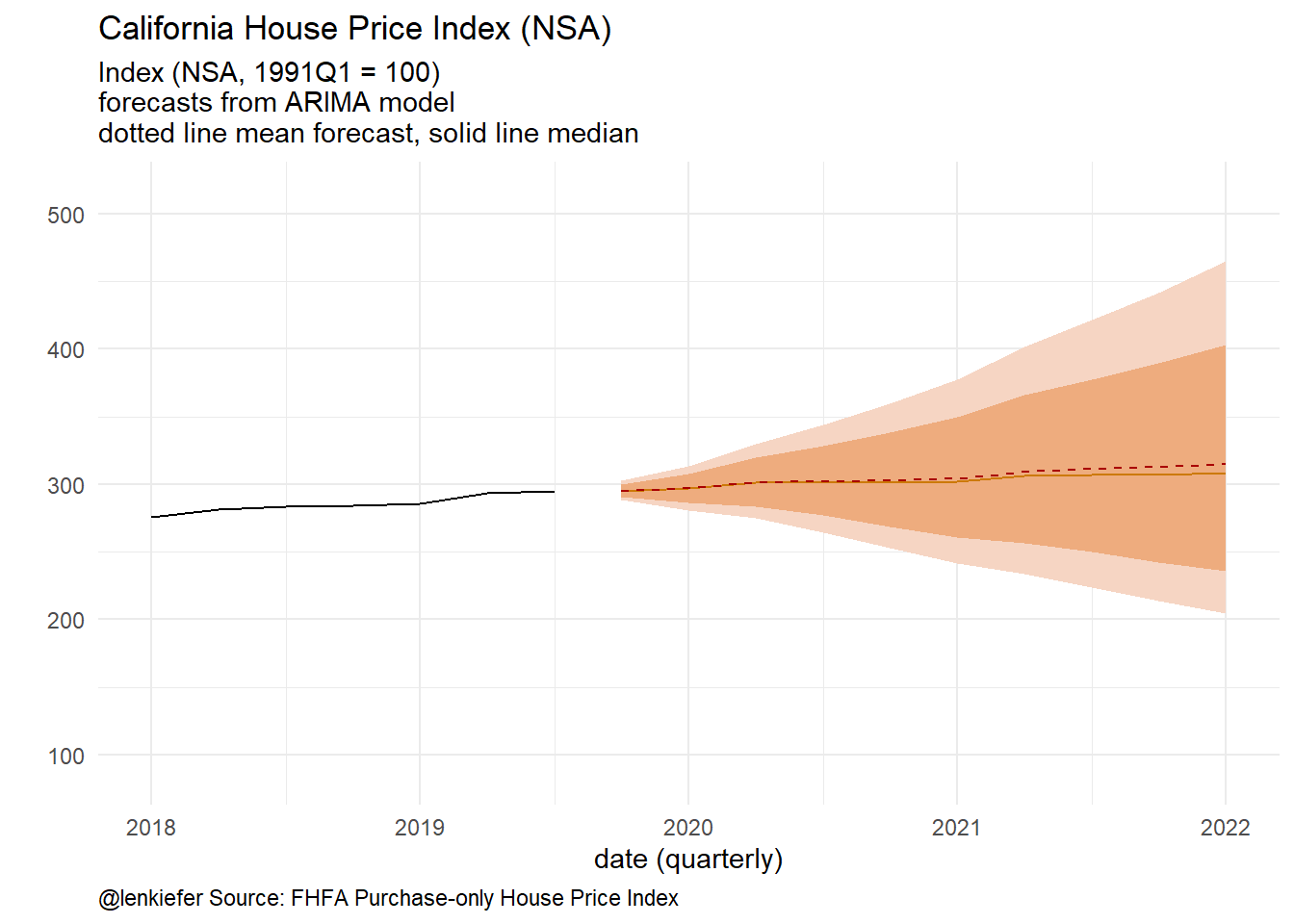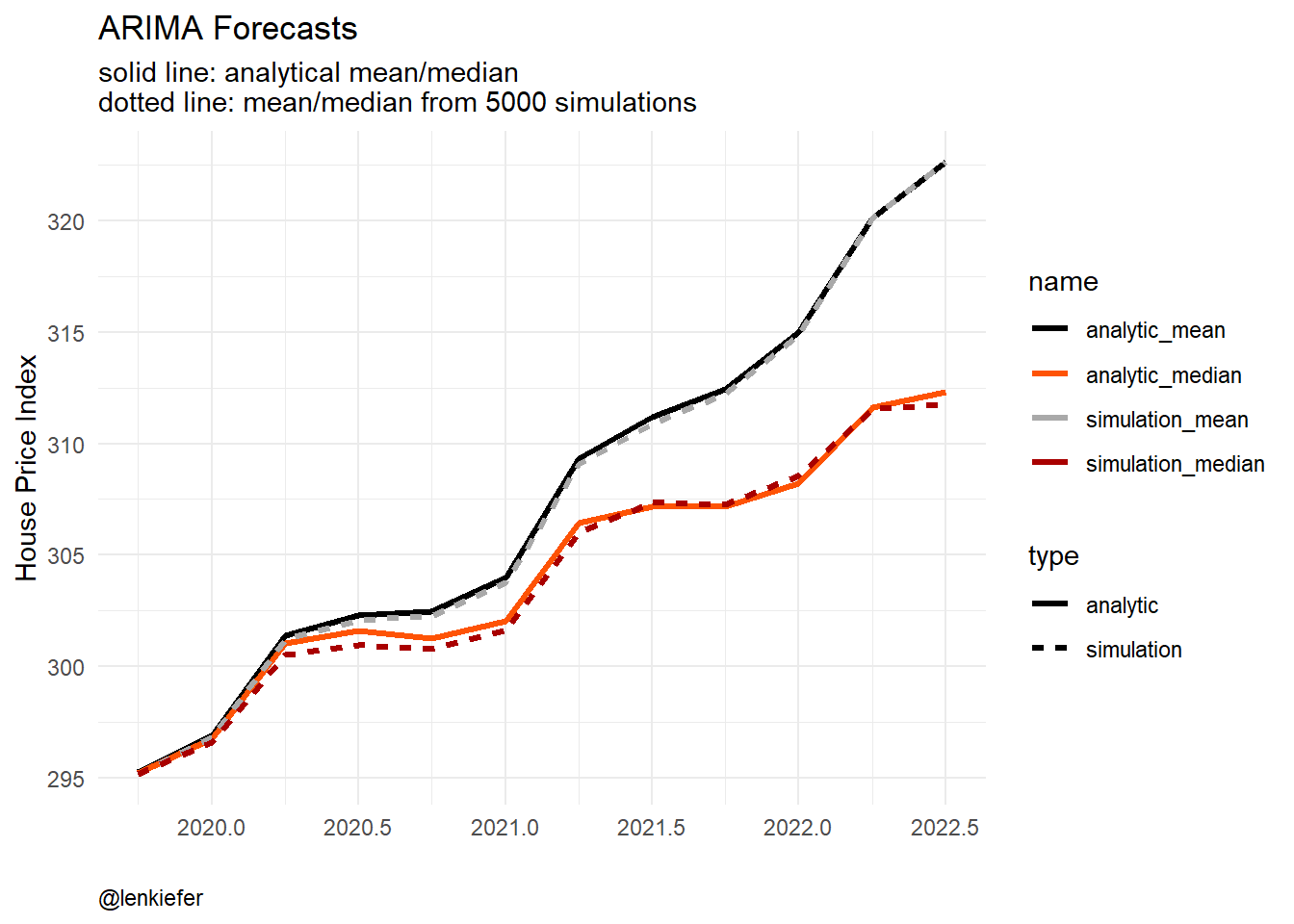# Forecasting with logs

## Notes on forecasting with logs

As an economist and all-around friend of strictly positive numbers I often use the log function. The natural logarithm of course, need I specify it? Apparently in certain spreadsheet software you do.

In this note I just wanted to write down a couple of observations about how to generate mean or median forecasts of a variable $$y$$ given the model is fit in $$log(y)$$. Of course, I am going to borrow heavily from Rob Hyndman’s blog, where he coverse this. But I also want to add in some code for a simulation from a seasonal arima model (also covered by Rob).

R code to follow

# A simple ARIMA model

Let’s fit a seasonal ARIMA model with drift to quarterly house price index for the state of California from the FHFA. The FHFA provides a nice flat text file that we can read straight into our computer:

#### Set up libraries

library(data.table)
library(tidyverse)
library(forecast)
library(matrixStats)
# colors (http://lenkiefer.com/2019/09/23/theme-inari/)
inari  <- "#fe5305"
inari1 <- "#e53a00"
inari2 <- "#a90000"

### Import data

# read in file

# construct  a times series
ts1 <- ts(dt[state=="CA",]$index_nsa ,start=1991,frequency=4) autoplot(ts1,color=inari,size=1.1)+ theme_minimal()+ theme(plot.caption=element_text(hjust=0))+ labs(x="date (quarterly)",y="", title="California House Price Index (NSA)", subtitle="Index (NSA, 1991Q1 = 100)", caption="@lenkiefer Source: FHFA Purchase-only House Price Index")Figure 1: House Price Index Let’s fit a simple seasonal ARIMA model to this index using forecast::auto.arima. Note this isn’t necessarily a great model, or useful for anything. It’s just a pretty standard benchmark. (fit <- auto.arima(ts1,lambda=0,allowdrift=TRUE)) ## Series: ts1 ## ARIMA(2,1,1)(2,0,0) ## Box Cox transformation: lambda= 0 ## ## Coefficients: ## ar1 ar2 ma1 sar1 sar2 ## 1.5855 -0.6934 -0.5263 0.3849 0.3375 ## s.e. 0.1522 0.1272 0.1824 0.0958 0.0965 ## ## sigma^2 estimated as 0.0001513: log likelihood=340.19 ## AIC=-668.38 AICc=-667.59 BIC=-651.96 fit1 <- Arima(ts1,model=fit) Construct foreasts: # forecast horizon = 12 quarters h <- 12 g1 <- autoplot(forecast(fit1,h),color=inari,size=1.1,fcol=inari)+ theme_minimal()+ theme(plot.caption=element_text(hjust=0))+ labs(x="date (quarterly)",y="", title="California House Price Index (NSA)", subtitle="Index (NSA, 1991Q1 = 100)\nforecasts from ARIMA model", caption="@lenkiefer Source: FHFA Purchase-only House Price Index") g1Figure 2: Forecasts from ARIMA Note that the default parameter setting for forecast::forecast has biasadj=FALSE, which means the default forecast (the line in the plot above) is the median. If we want the mean forecast we can set biasadj=TRUE or just do it ourselves: fc <- forecast(fit,h, level=95) # as in https://robjhyndman.com/hyndsight/backtransforming/ fvar <- ((BoxCox(fc$upper,fit$lambda) - BoxCox(fc$lower,fit$lambda))/qnorm(0.975)/2)^2 fc$mean_log <- fc$mean * (1+0.5*fvar) colnames(fc$mean_log) <-  "mean_log"
g1+autolayer(fc$mean_log, linetype=2,color=inari2)+scale_x_continuous(limits=c(2018,2022))+ labs( subtitle="Index (NSA, 1991Q1 = 100)\nforecasts from ARIMA model\ndotted line mean forecast, solid line median")Figure 3: Mean and median forecasts # A simulation It’s always nice to check our results against a simulation. # simulate from ARIMA https://robjhyndman.com/hyndsight/simulating-from-a-specified-seasonal-arima-model/ Nsim=5000 h <- 12 ts_sim=array(dim=c(Nsim,h)) for (i in 1:Nsim){ ts_sim[i,] <- simulate(fit1,nsim=h) } # create ts object ts_sim <- ts(t(ts_sim),start=start(fc$mean),frequency=frequency(fc$mean)) colnames(ts_sim) <- paste0("sim_",1:Nsim) # construct simulation means and medians fc_median <- ts(rowMedians(ts_sim),start=start(fc$mean),frequency=frequency(fc$mean)) fc_mean <- ts(rowMeans(ts_sim),start=start(fc$mean),frequency=frequency(fc$mean)) And make a plot: # combine into data frame/tibble df_plot <- tibble(tt=as.numeric(time(fc$mean)),
analytic_median=fc$mean, simulation_median=fc_median, simulation_mean=fc_mean, analytic_mean=fc$mean_log[,1])

# make plot
df_plot %>%
pivot_longer(-tt) %>%
mutate(type=ifelse(grepl("sim",name),"simulation","analytic")) %>%
ggplot(aes(x=tt,y=value,color=name,linetype=type))+
geom_line(size=1.1)+
scale_color_manual(values=c("black",inari,"darkgray",inari2))+
theme_minimal()+
theme(plot.caption=element_text(hjust=0))+
labs(x="",y="House Price Index",
title="ARIMA Forecasts",
subtitle="solid line: analytical mean/median\ndotted line: mean/median from 5000 simulations",
caption="@lenkiefer")Figure 4: Simulation Comparison

As time series go this is pretty straightforward stuff. I am just putting this down here so future me can reference this as needed.